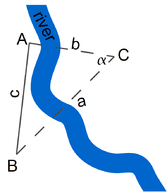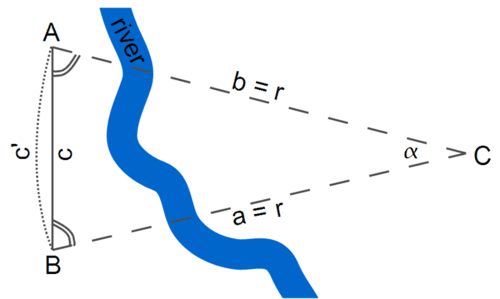# 18.1: Pre-Trigonometry

•• Contributed by CK12
• CK12Figure $$\PageIndex{1}$$: The basic problem of trigonometry.

The page at http://www.phy6.org/stargaze/Strig1.htm describes the basic problem of trigonometry (drawing above): finding the distance to some far-away point C, given the directions at which C appears from the two ends of a measured baseline AB. This problem becomes somewhat simpler if:

1. The baseline is perpendicular to the line from its middle to the object, so that $$\Delta ABC$$ is symmetric. We will denote its equal sides as $$AB=BC=r$$.
2. The length $$c$$ of the baseline $$AB$$ is much smaller than $$r$$. This means that the angle $$\alpha$$ between $$AC$$ and $$BC$$ is small; that angle is known as the parallax of $$C$$, as viewed from $$AB$$.
3. We do not ask for great accuracy, but are satisfied with an approximate value of the distance --- say, within 1%.Figure $$\PageIndex{2}$$: A simplified version of the problem (not to scale).

The method presented here was already used by the ancient Greeks more than 2000 years ago. They knew that the length of a circle of radius $$r$$ was $$2\pi r$$, where $$\pi$$ (a modern notation, not one of the Greeks, even though $$\pi$$ is part of their alphabet) stands for a number a little larger than 3; approximately

$$\pi\approx\,3.14159\ldots$$

Note

The Greek mathematician Archimedes derived $$\pi$$ to about 4-figure accuracy, though he expressed it differently, since decimal fractions appeared in Europe only some 1000 years later.

In this case (see Figure above), we can approximate$$\Delta ABC$$ as a ‘slice’ of a much bigger circle; in this case, the length of the baseline is approximately equal to the length of the corresponding arc:

$c\,\approx\,c′$

There are 360 degrees in a circle and$$\alpha$$ degrees in this particular arc; since 360 degrees corresponds to one circumference of arc length ($$2\pi r$$), $$\alpha$$ degrees will correspond to an arclength of

$c′\,=\,\frac{\alpha}{360^o}\times 2\pi r$

Solving for $$r$$ and plugging in $$c\,\approx\,c′$$, we find

$r\,=\,\frac{360^o}{2\pi\alpha}\times c$

We have solved for $$r$$ in terms of $$c$$. For instance, if we know that $$\alpha\approx 6^o$$ (we'll see why this is relevant later), $$2\pi\alpha\,=\,36^o$$ and we get:

$r\,=\,10c′$